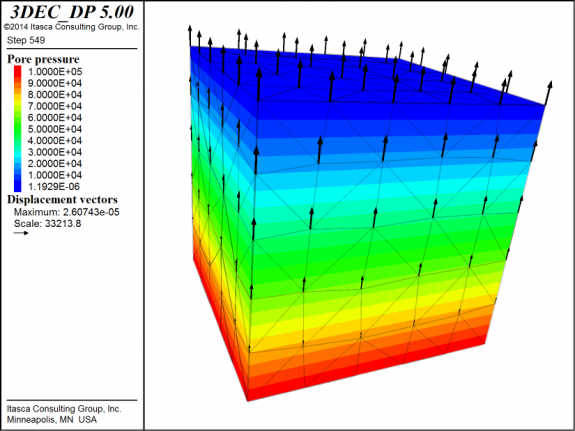## Adjust Total Stress in 3DEC

3DEC includes the ability to simulate fluid flow through joints. The calculations are fully coupled such that pressure changes can cause displacements of the solid. However, often we don’t want to deal with the complexity of modelling fluid flow through joints, or even modelling fluid flow at all.

3DEC also therefore provides a much simpler approach to considering pore pressures. A water table can be specified (as a simple plane, or an imported surface geometry) and pore pressures are calculated at the centroid of each zone based on the depth below the water table, the water density and gravity. The pore pressures are then used to calculate effective stresses in the zones. The effective stress is then used when checking if the material strength has been exceeded.

Unlike in UDEC, pore pressure changes have no effect on the total stress in 3DEC. What this means is that if the water table is raised or lowered, no deformation will be observed (unless material strength has been exceeded). To account for changes in total stress, the user must use a FISH function to manually change the zone stresses based on pore pressure changes.

The plots below show a simple block that is initially dry. The water table is then raised to the top of the block. By using the fish functions to adjust the total stress, you can see the heave of the material due to the increased pore pressures. The data file and fish functions to execute this example are given here: ats.zip